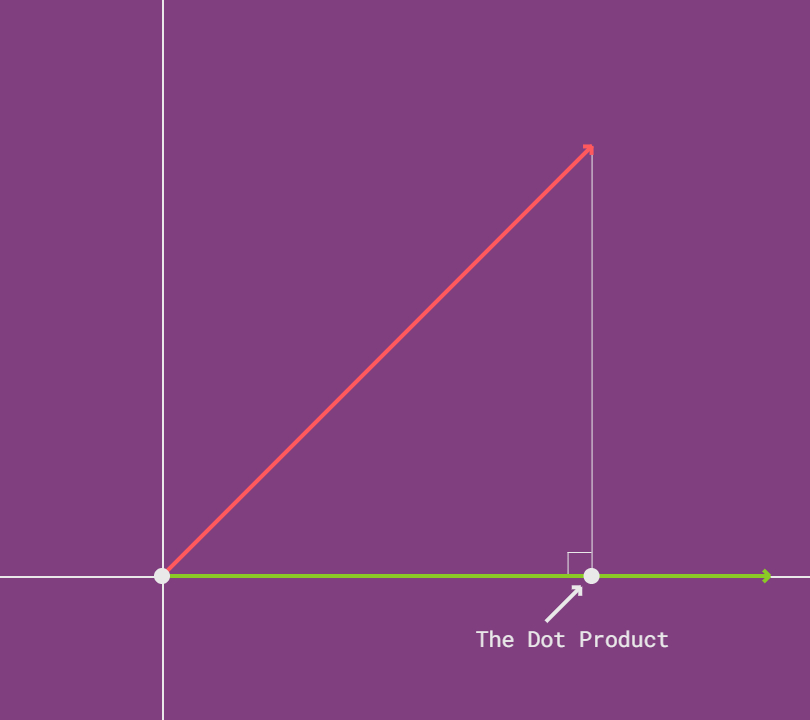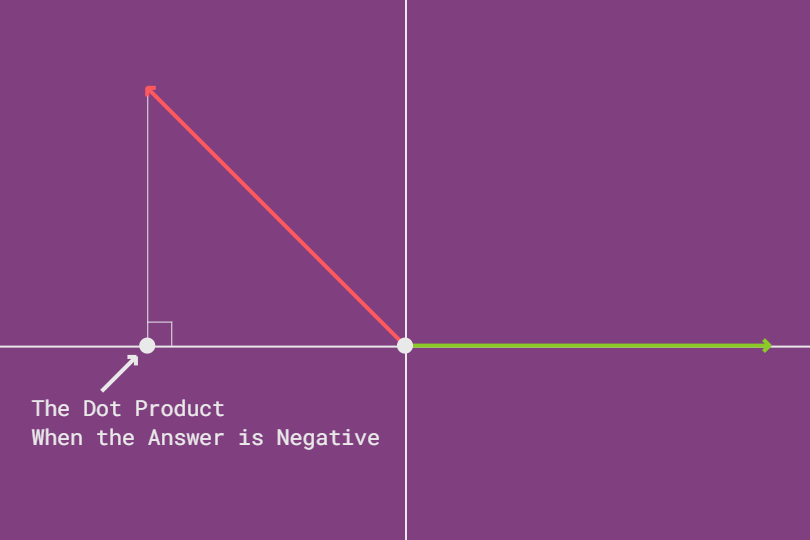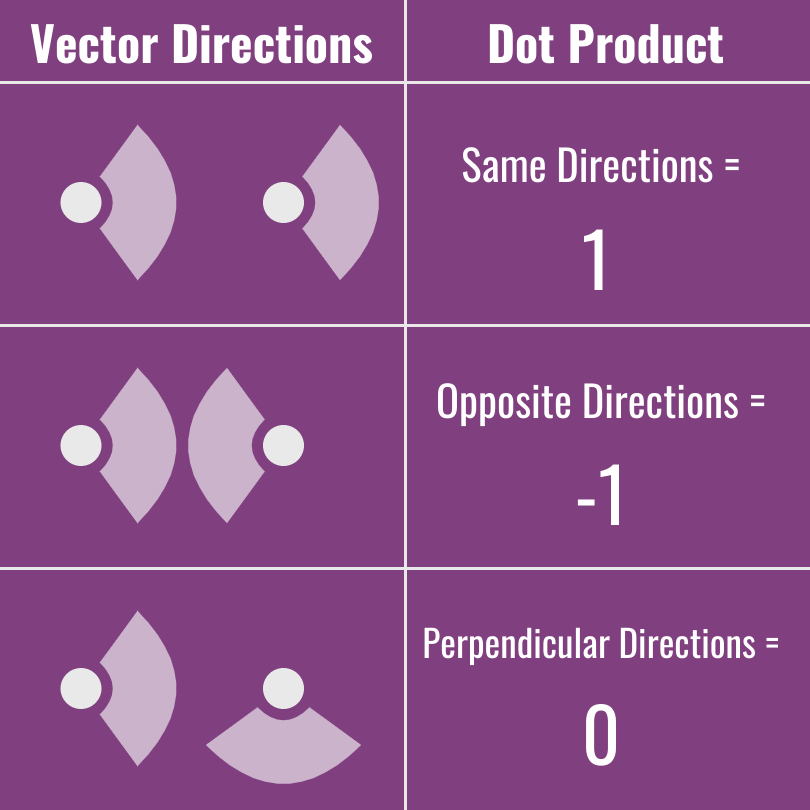# Interactive Dot Product of Two Vectors

We use the dot product of two vectors to answer many questions.

What kind of questions?
Let's say that we make a game and we need to answer the following:
• Are two characters looking in the same direction?
• Is a character looking at a sign?
• How should a ray of light interact with a surface?
We answer these types of questions with the dot product.

### What Is the Dot Product

The dot product is an operation that takes in two vectors and returns a number.
That description probably doesn't help much.
The dot product tells us how similar the directions of our two vectors are.
Remember that a vector is a length and direction;
a vector tells us how far to move in it's direction.

### How to Find the Dot Product

Let's say that we have two vectors named `vector A `and `vector B. `
There are two ways we can find the dot product of our vectors.

### Way #1: With Basic Algebra

We think of this as "the developer way" to find the dot product. `A.x * B.x + A.y * B.y` Simple, no? Only multiplication and addition. Superfast for computers to do.

This way makes us do more work to normalize our dot product, which, in this case, means we want our result in the range of -1 to 1.
If we want to convert a number into one, we divide that number by itself; So, we divide by the lengths of both of our vectors multiplied together.
`A.x * B.x + A.y * B.y / (||A|| * ||B||)` The `||` around our vectors mean we're taking their length. We use two bars because one could mean we're taking the absolute value.

### Way #2: With Basic Trig

We think of this as the "pure math" way.
We subtract the angle of one of our vectors from the angle of the other (works either way).
We then take the cosine of the result or our subtraction.
We then multiply the result of our cosine by the magnitude (length) of both of our vectors.
Viola, we have our dot product. `cos(Angle A - Angle B) * ||A|| * ||B||` Our case is simpler, because we're interested in our result being normalized (we want our result in the range of -1 to 1).

Hmm that's the range of cos...
`cos(Angle A - Angle B)` That's all we need, our normalized dot product is the cosine of the difference of the angles of our vectors...interesting.

### Which Way Is the Best?

So, how do we know which way to use?
It depends on which data we have.
If we already have the positions, we can use the algebra way.
If we already have the angles, we can use the trig way.
If we have neither, we will get whichever is easiest for us.
Both ways give us the same result! Notice how both ways have an element of directionality.

### Dot Product Projection

We think of the dot products as a projection of one vector onto the other.
The idea is simple.
We draw both of our vectors, then drop a line from the tip of one to connect them with a 90° angle.Figure A

We notice that our result is the cosine of the triangle we created when we dropped our line.

What happens when the vectors don't overlap?
If the vectors don't overlap we would have a negative result; We "extend" the vector we are projecting on in the opposite direction, then drop our line.Figure B

### The Scalar Product

Do you think it's strange that the dot product takes in two vectors but doesn't return a vector?

Instead, it returns a number, which we could call a scalar.
This is why some call the dot product the scalar product.

Different name but the same thing.

Why does it make sense that the dot product returns a scalar and not a new vector?
Because the dot product is describing the relationship between two vectors.

### The Inner Product Vs the Dot Product

The inner product is more general than the dot product. The dot product is a special case of the inner product. That is, the dot product is an application of the inner product, but the inner product goes beyond the dot product.

### What Does the Dot Product Represent?

We get a number(scalar), not a vector, as a result; The result describes the relationship between the two vectors. The closer the result is to 1 the more similar the vectors are, the closer the result is to -1 the more dissimilar, the closer the result is to 0 the closer two vectors are to being perpendicular.Figure C

### Conclusion

The dot product answers the question: how similar are the directions of two vectors.
The answer to this question solves many different types of problems for us.